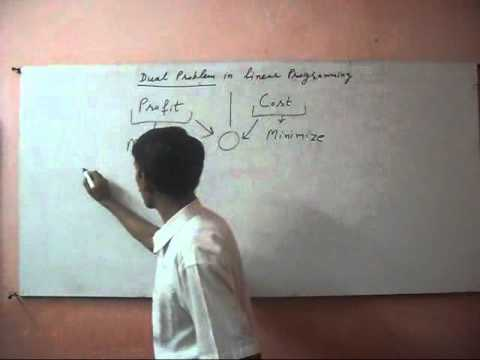# Why SvTuition Important# Why SvTuition Volunteer## Become Volunteer Teacher

Linear programming is the advance technique of effective utilization of resources. With financial mathematics, we can convert our financial problem in to equation type problem or linear programming problem. Dual problem is the same problem of original problem. If we say, we have to maximize our profit, it means, we are saying that we have to minimize our cost. We can easily convert profit maximization equation into cost minimization equation.  Above video tutorial will explain it deeply.## Ashok Kumar's Contents\$type=blogging\$show=https://www.svtuition.com/p/ashok-kumar.html\$hide=author

Name

ltr
item
Svtuition: Dual Problem in Linear Programming
Dual Problem in Linear Programming
https://i.ytimg.com/vi/Uy1is1ev74M/hqdefault.jpg
https://i.ytimg.com/vi/Uy1is1ev74M/default.jpg
Svtuition
https://www.svtuition.com/2012/06/dual-problem-in-linear-programming.html
https://www.svtuition.com/
http://www.svtuition.com/
http://www.svtuition.com/2012/06/dual-problem-in-linear-programming.html
true
3065238051582539150
UTF-8